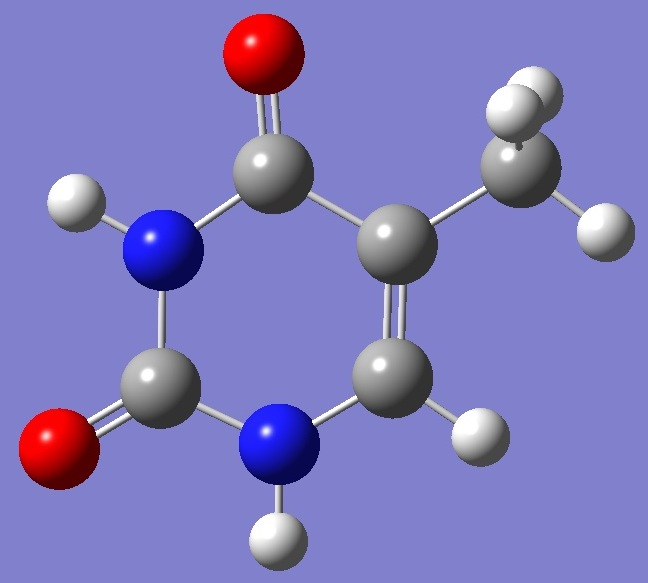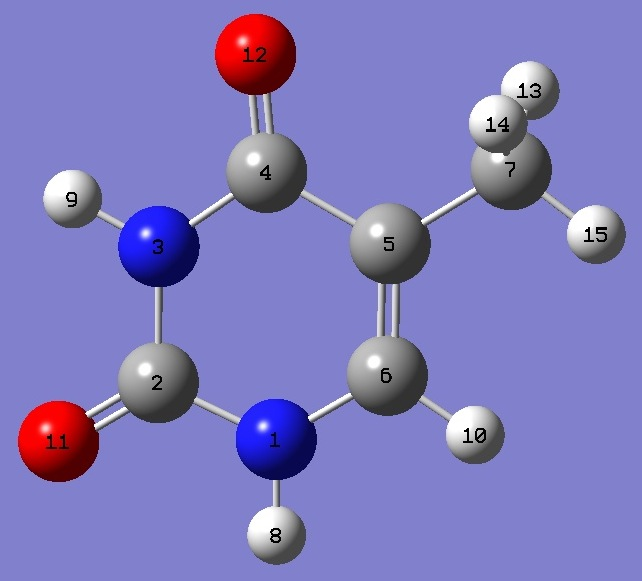ThymineNitrogen

Nuclear Quadrupole Coupling Constants

in Thymine

14N nqcc's in thymine were determined by López et al. .   A semi-experimental equilibrium structure (reSE) was derived by Vogt et al. .

Calculation of the nqcc's was made here on the reSE structure.  These calculated nqcc's are compared with the experimental values in Tables 1 and 2.  Structure parameters are given in Z-matrix format in Table 3.

Calculation was made with both B3PW91/6-311+G(df,pd) and B3PW91/6-311+G(d,p) models.

In Tables 1 and 2, subscripts a,b,c refer to the principal axes of the inertia tensor, subscripts x,y,z to the principal axes of the nqcc tensor.  The z-principal axis of the nqcc tensor is perpendicular to the symmetry plane of the molecule.  ETA = (Xxx - Xyy)/Xzz.

RMS is the root mean square difference between calculated and experimental nqcc's (percentage of average experimental nqcc).  RSD is the residual standard deviation of calibration of the model used for calculation of the efg's/nqcc's.

 Table 1.  14N(1) (see figure below) nqcc's Thymine (MHz).  Calculation was made on the reSE structure with (1) B3PW91/6-311+G(df,pd) and (2) B3PW91/6-311+G(d,p) models. Calc (1) Calc (2) Expt.  Xaa 1.845 1.931 1.954(11) Xbb 1.782 1.860 1.850(12) Xcc - 3.627 - 3.791 - 3.804(12) |Xab| 0.147 0.169 RMS 0.126 (4.5 %) 0.016 (0.62 %) RSD 0.030 (1.3 %) 0.086 (3.8 %) Xxx 1.964 2.069 Xyy 1.663 1.722 Xzz - 3.627 - 3.791 - 3.804(12) ETA - 0.0830 - 0.0914

 Table 2.  14N(3) (see figure below) nqcc's Thymine (MHz).  Calculation was made on the reSE structure with (1) B3PW91/6-311+G(df,pd) and (2) B3PW91/6-311+G(d,p) models. Calc (1) Calc (2) Expt.  Xaa 1.713 1.807 1.768(13) Xbb 1.627 1.687 1.652(14) Xcc - 3.340 - 3.494 - 3.420(14) |Xab| 0.213 0.230 RMS 0.058 (2.5 %) 0.052 (2.5 %) RSD 0.030 (1.3 %) 0.086 (3.8 %) Xxx 1.888 1.984 Xyy 1.452 1.510 Xzz - 3.340 - 3.494 - 3.420(14) ETA - 0.130 - 0.136

 Table 3.  Thymine.  Molecular structure parameters, reSE (Å and degrees).  Z-matrix for reSE structure was derived here from coordinates given in Table 5 of Ref. .N C,1,B1 N,2,B2,1,A1 C,3,B3,2,A2,1,D1,0 C,4,B4,3,A3,2,D2,0 C,5,B5,4,A4,3,D3,0 C,5,B6,4,A5,3,D4,0 H,1,B7,6,A6,5,D5,0 H,3,B8,2,A7,1,D6,0 O,2,B9,1,A8,6,D7,0 O,4,B10,3,A9,2,D8,0 H,6,B11,5,A10,4,D9,0 H,7,B12,5,A11,4,D10,0 H,7,B13,5,A12,4,D11,0 H,7,B14,5,A13,4,D12,0 B1=1.37817543 B2=1.37968081 B3=1.39319951 B4=1.46349869 B5=1.34570239 B6=1.49301575 B7=1.00386099 B8=1.00868418 B9=1.20948554 B10=1.21532214 B11=1.08039770 B12=1.08835754 B13=1.08929505 B14=1.08929505 A1=112.77075237 A2=127.96721549 A3=114.80580484 A4=117.87768474 A5=117.83616603 A6=120.94781756 A7=115.56872554 A8=123.38751815 A9=120.42085926 A10=122.16444720 A11=110.77063946 A12=110.56536854 A13=110.56536854 D1=0. D2=0. D3=0. D4=180. D5=180. D6=180. D7=180. D8=180. D9=180. D10=180. D11=59.19638655 D12=-59.19638655

 J.C.López, M.I.Peña, M.E.Sanz, and J.L.Alonso, J.Chem.Phys. 126,191103(2007).

 N.Vogt, J.Demaison, D.N.Ksenafontov, and H.D.Rudolph, J.Mol.Struct. 1076,483(2014).

Uracil
2-Pyridone

Molecules/Nitrogen

thymine.html